## ECE 564 Computer Vision Assignment 6

Submit the usual HTML documentation and source code on Isidore.

Use the photographs from CV2020-02-05 and CV2020-02-24.

1. Given a triangle represented by three principal vanishing points, write a MATLAB code to determine the orthocenter and distances d1 and d2 for each vertex. Check that the focal length formula gives the same result for each case.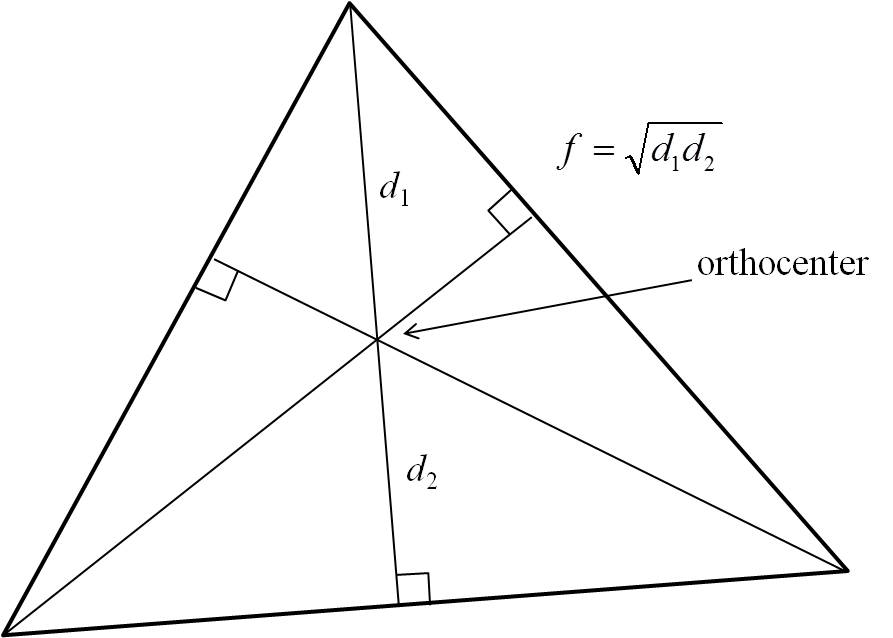2. Write a MATLAB function to determine the Euler line of a triangle. Demonstrate that the three centers do in fact lie on the Euler line (see references)

3. Use CV2020-02-05 showing the target board on floor, right-wall, and left-wall.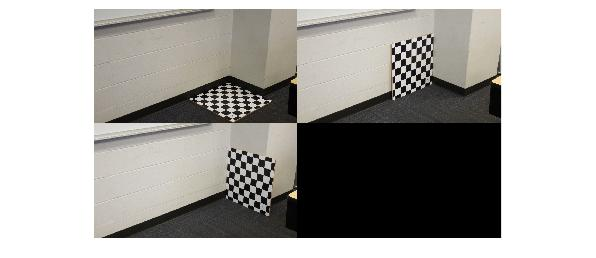1. Find the three principal horizon lines and construct an “ortho triangle” to determine the orthocenter (principal point) and focal length of the camera. Compare to the MATLAB camera calibration you performed in an earlier assignment.

2. Construct a diagram similar to the example below.4. Use the first hall image from CV2020_05_24 (see below) and camera calibration data obtained earlier to find the the location of the camera and checkerboard relative to the hallway. Find the height of the camera above the floor. Calculate the “look-down” angles of the camera. Use the photographs taken of the camera to obtain another measure of the “look-down” angle.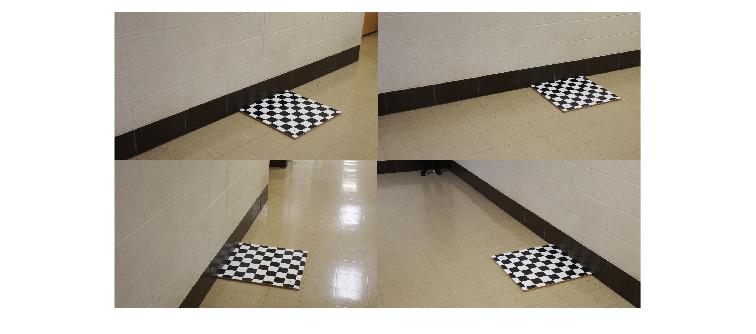5. Construct a `tform` to show a top-view of the hallway from the top-left image above. Use the same `tform` to warp the other images. Does the checkerboard look square in these other images?

6. Use the same `tform` for the images below. If the `tform` is inapproriate, generate a new one from the top-level image below. Calculate the width of the hallway and the divergence angle of the hallway.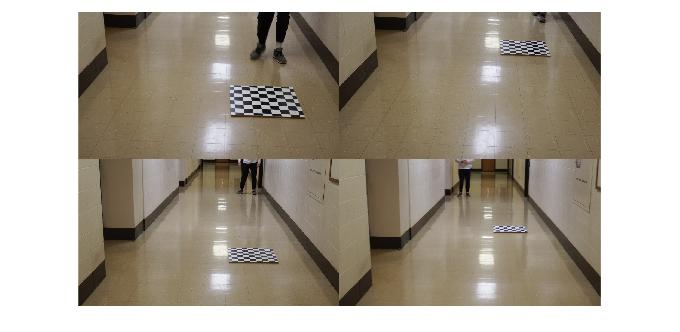7. Construct a layout drawing similar to the one shown below, showing the geometry of the hallway, the position and orientation of the camera and the bottom of the camera field of view. The asterisk shows the camera target point (projection of camera z-axis to the transformed image).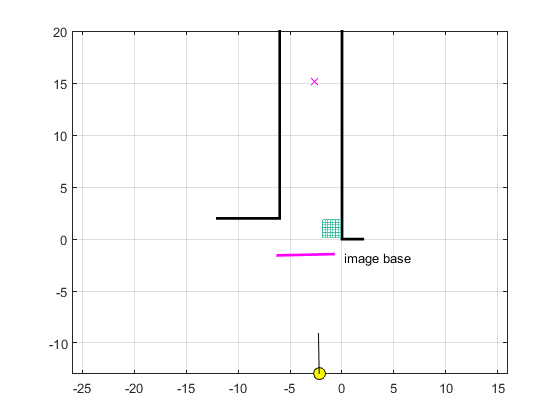### References

Orthocenter of a triangle (Wikipedia)
Orthocenter (with animation) (Math Open Reference)
Euler line (Wikipedia)
Euler line (Math Open Reference)